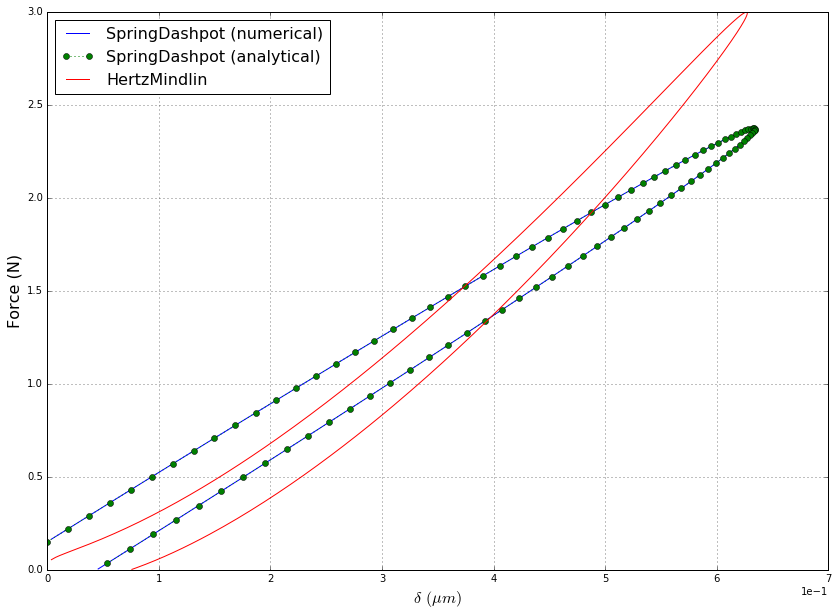# Numerical Analysis of Contact Models¶

In this tutorial, we compute the force-displacement curves for 2 different viscoelastic models: Spring-Dashpot and Hertz-Mindlin. The input script file is found in the examples/scripts/Contact-Models directory.

Since visco-elastic models suffer from an unphysical attractive force at the end of the contact, we supply the 'limitForce' boolean variable to the contact model in order to ensure any attractive forces at the end of the contact are ignored.

In [ ]:
# Import simulation module
from pygran import simulation as sim

# Import material propreties for steel
from pygran.params import steel

# Import matplotlib for plotting
import matplotlib.pyplot as plt

# Create a list of contact models to analyze
models = [sim.models.SpringDashpot, sim.models.HertzMindlin]

# Set particle radius to 1 mm
plt.figure(figsize=(14,10))

# Compute the force-displacement curve
for model in models:
model = model(material=steel, limitForce=True)

time, delta, force = model.displacement()
plt.plot(delta[:,0] * 1e6, force)

if hasattr(model, 'displacementAnalytical'):
time, delta, force = model.displacementAnalytical()
plt.plot(delta[:,0] * 1e6, force, ':o')